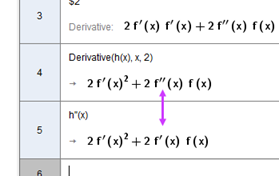# Bug when calculating the derivative in CAS-mode.

Jakob Jakobsson shared this question 5 years ago

When I write as follows:

h(x):=(f(x))^2

h''(x)

I get the answer: 2f'(x)² + 2f'(x) f(x). But the correct answer is 2f'(x)² + 2f''(x) f(x)

If I use the button f' two times then I get the correct answer.1

Confirmed in Classic v.5 and v. 61

isn't the following result correct? (without any plus sign...)

chris1

Hi Chris. I don't understand your CAS window.

Did you type just h(x) and press Enter in the 2nd row? If I do the same on online Classic, it yields f(x)^2...1

hi Simona two ways to get the solution (in GG6):

in row 1 I typed (f(x))² and then I clicked on the derivate tool.

in row 2 I defined h(x):=(f(x))² and entered, as you thought I might have. Then row 3 opens and I only have to click on the derivate tool to get the result.

chris1

the problem is in second derivative1

disculpe

the extra comma for the second derivate escaped to me... Strange that you can be so focussed on what you think to see that you even don't get the link between the formula and the obvious second derivate.

chris1

No worries :)

I've handed over the hot potato to the Team1

Thanks, fixed for next release!1

Please try the new version (387)